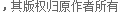# 织梦DedeCMS模板可以使用的公共函数集合_DedeCms教程

function GetCurUrl() //获得当前的脚本网址
function MyDate(\$format='Y-m-d H:i:s',\$timest=0) //返回格林威治标准时间
function GetAlabNum(\$fnum) //把全角数字转为半角
function Html2Text(\$str,\$r=0) //把含HTML的内容转为纯text
function Text2Html(\$txt) //把文本转HTML
function cn_substr(\$str,\$slen,\$startdd=0) //中文截取2，单字节截取模式
function GetMkTime(\$dtime) //把标准时间转为Unix时间戳
function GetDateTimeMk(\$mktime)//获得一个 0000-00-00 00:00:00 标准格式的时间
function GetDateMk(\$mktime) //获得一个 0000-00-00 标准格式的日期
function GetIP() //获得用户IP
function ShowMsg(\$msg,\$gourl,\$onlymsg=0,\$limittime=0) //dedecms通用消息提示框
function GetCkVdValue() //获取验证码
function HtmlReplace(\$str,\$rptype=0)
//过滤前台用户输入的文本内容
// \$rptype = 0 表示仅替换 html标记
// \$rptype = 1 表示替换 html标记同时去除连续空白字符
// \$rptype = 2 表示替换 html标记同时去除所有空白字符
// \$rptype = -1 表示仅替换 html危险的标记
function GetTags(\$aid) //获得某文档的所有tag
function FilterSearch(\$keyword) //过滤用于搜索的字符串
function TrimMsg(\$msg) //处理禁用HTML但允许换行的内容
function GetOneArchive(\$aid) //获取单篇文档信息
function utf82gb(\$utfstr) //UTF8编码转换为GBK编码
function gb2utf8(\$gbstr) //GBK编码转换为UTF8编码
function dd2char(\$ddnum) //生成一个随机字符
function json_encode(\$data) //对变量进行JSON编码
function json_decode(\$json, \$assoc=FALSE) //对变量进行JSON解码

• 相关链接：
• 教程说明：

DedeCms教程-织梦DedeCMS模板可以使用的公共函数集合